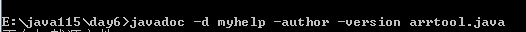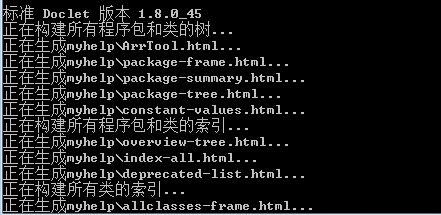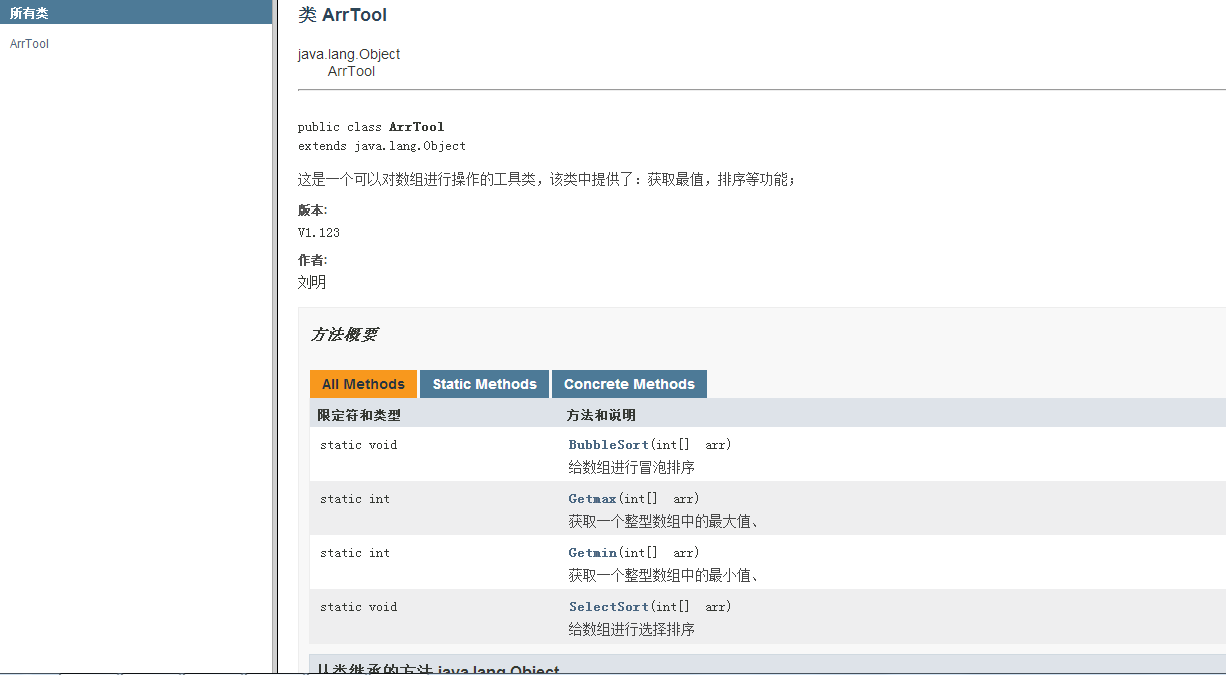# 帮助文档的制作（程序说明书）

/**

@author 刘明
@version V1.123
*/

pulic class ArrTool//必须用public修饰
{
/**
空参数构造函数
*/
private ArrTool(){}
/**
获取一个整型数组中的最大值、
@param（参数）  arr 接收一个int类型的数组//（参数）是方便此次阅读，制作时不能写，一写就报错；
@return 会返回一个该数组的最大值
*/
public static int Getmax(int[] arr)
{
int max=0;
for(int x=0;x<arr.length;x++)
{
if(arr[x]>arr[max])
max=x;
}
return arr[max];
}
/**
获取一个整型数组中的最小值、
@param（参数）  arr 接收一个int类型的数组
@return 会返回一个该数组的最小值
*/
public static int Getmin(int[] arr)
{
int min=0;
for(int x=0;x<arr.length;x++)
{
if(arr[x]<arr[min])
min=x;
}
return arr[min];
}
/**
给数组进行选择排序
@param（参数）  arr 接收一个int类型的数组
*/
public static void SelectSort(int[] arr)
{
for(int x=0;x<arr.length-1;x++)
{
for(int y=x+1;y<arr.length;y++)
if(arr[x]>arr[y])
{
Swap(arr,x,y);
}
}
}
/**
给数组进行冒泡排序
@param（参数）  arr 接收一个int类型的数组
*/
public static void BubbleSort(int[] arr)
{
for(int x=1;x<arr.length-1;x++)
{
for(int y=0;y<arr.length-x;y++)
{
if(arr[y]>arr[y+1])
Swap(arr,y,y+1);
}
}
}
/**
给数组中的元素进行位置置换。
@param arr 接收一个int类型的数组。
@param a  要置换的位置。
@param  b  要置换的位置。
*/
private static void Swap(int[] arr,int a,int b)
{
int t=arr[a];
arr[a]=arr[b];
arr[b]=t;
}
}-d表示保存到文件夹，后面跟文件夹地址，myhelp,如果当前目录没此文件夹则会自动创建，当然也可以创建在C盘（C:\myhelp）；

-author -version 时显示作者和版本的，可写可不写，根据自己要求；12-23
02-25
03-101156
11-10117
11-214万+
02-02
10-231458
07-034356
10-168
09-25
01-1760
01-07
10-11
©️2020 CSDN 皮肤主题: 大白 设计师:CSDN官方博客